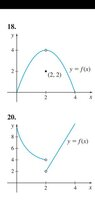# Investigating A Limit Via Graph

Homework Statement:
Graphs and Limits
Relevant Equations:
Use the graph to investigate

(a) lim of f(x) as x→2 from the left side.

(b) lim of f(x) as x→2 from the right side.

(c) lim of f(x) as x→2.

Question 18

For part (a), as I travel along on the x-axis coming from the left, the graph reaches a height of 4. The limit is 4. It does not matter if there is a hole at (2, 4), right?

For part (b), as I travel along on the x-axis coming from the right, the graph reaches a height of 4. The limit is 4. It does not matter if there is a hole at (2, 4), right?

For part (c), as I travel along on the x-axis coming from the left and right simultaneously, the graph reaches a height of 4. The limit is 4. It does not matter if there is a hole at (2, 4), right?

I conclude that the limit is 4.

You say?

#### Attachments

•Screenshot_20210613-160046_Drive.jpg
9.6 KB · Views: 45

Mentor
Question 18

For part (a), as I travel along on the x-axis coming from the left, the graph reaches a height of 4. The limit is 4. It does not matter if there is a hole at (2, 4), right?
Correct. The left-side limit if 4, and the presence of a hole doesn't matter.
nycmathguy said:
For part (b), as I travel along on the x-axis coming from the right, the graph reaches a height of 4. The limit is 4. It does not matter if there is a hole at (2, 4), right?
Correct. The right-side limit if 4, and the presence of a hole doesn't matter.
nycmathguy said:
For part (c), as I travel along on the x-axis coming from the left and right simultaneously, the graph reaches a height of 4. The limit is 4. It does not matter if there is a hole at (2, 4), right?

I conclude that the limit is 4.
Yes, correct.

•nycmathguy
Correct. The left-side limit if 4, and the presence of a hole doesn't matter.
Correct. The right-side limit if 4, and the presence of a hole doesn't matter.
Yes, correct.

I finally get one right on my own.